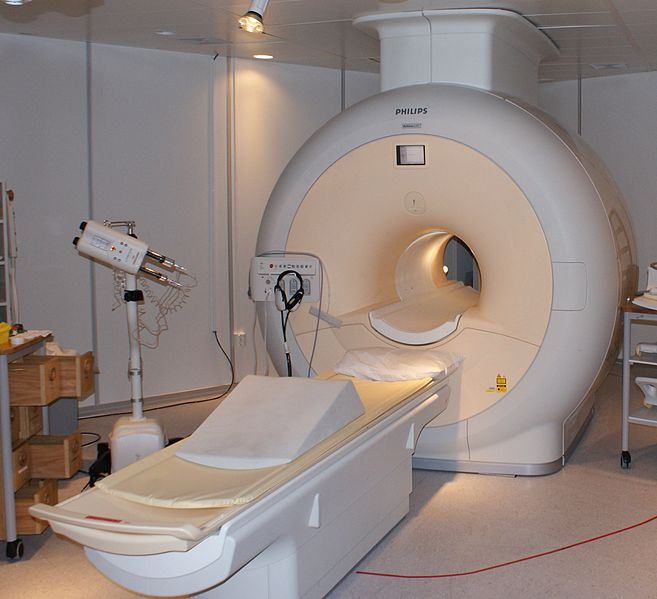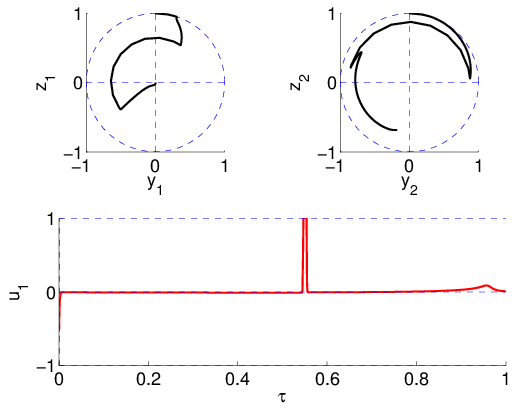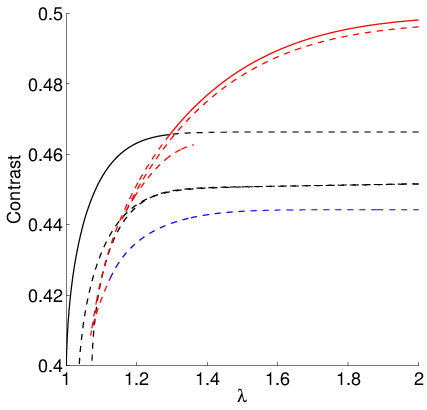# Contrast in magnetic resonance imaging (MRI)Magnetic Resonance Imaging (MRI) is a medical imagery technique that does not expose the body to ionizing radiation (such as X-ray). Instead, it relies on a strong magnetic field to excite atoms in the tissues, more  specifically hydrogen atoms present in water (water accounts for 70% of the human body mass). Measuring the rate at which the atoms go back to their equilibrium state allows to reconstruct the spatial distribution of water, and by extension to differentiate tissue types.Finding the magnetic field that maximizes the contrast between two types of tissue can be written as an optimal control problem, studied for instance in [1,2]. The magnetization vector$Latex formula$ for each 1/2 spin particle follows the Bloch equation (here in the 3D, bi-input case)$Latex formula$

with u the magnetic field (control) and$Latex formula$ relaxation parameters depending on the tissue. In the simplified, 2D, mono-input case, we get$Latex formula$

Considering two different particles with spins$Latex formula$, the contrast is  linked to$Latex formula$ at the end of the excitation phase. The classical “contrast by saturation” method consists in bringing one  spin to the origin (saturation) and the other as far as possible. Assuming both spins start from the equilibrium point at the north pole, the optimal control problem is$Latex formula$

with the final time set to a multiple of the minimum time$Latex formula$ for the saturation of$Latex formula$. The Hamiltonian is linear in the control, and it can be shown that the optimal control is a sequence of Bang and Singular arcs, noted nBS (ie 2BS denotes a bang-singular-bang-singular structure).

We illustrate this problem on the (cerebro-spinal fluid,water) case, see below, with an example of a 2BS structure.MRI: contrast by saturation, (cerebro-spinal fluid,water) case.

A comprehensive study using indirect shooting and differential continuation methods (HAMPATH,) indicates the existence of numerous families of local solutions with  different structures, and shows that the optimal structure depends on the final time, as shown here.Contrast: branches of local solutions (1BS: black, 2BS: blue, 3BS: red)

References

 B. Bonnard, M. Claeys, O. Cots and P. Martinon. Comparison of Numerical Methods in the Contrast Imaging Problem in NMR. In 52nd IEEE Conference on Decision and Control, Firenze, Italy, 2013.

 B. Bonnard, M. Claeys, O. Cots and P. Martinon. Geometric and numerical methods in the contrast imaging problem in the contrast imaging problem in nuclear magnetic resonance. Acta Applicandae Mathematicae, pages 1–41, 2015.

 O. Cots. Controle optimal geometrique: methodes homotopiques et applications. PhD thesis, 2012.